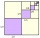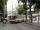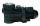# Quadratic equation - problems

1. Loan 5Abdul takes a loan of 200000 from Ali and agrees to repay in number of instalment, each instalment begin with the 2nd exceeding the previous one by 1000, if the first instalment is 500, find how many instalment will be necessary to be wipe out the loan? C
2. Find parametersFind parameters of the circle in the plane - coordinates of center and radius: ?
3. 3rd dimensionThe block has a surface of 42 dm2 and its dimensions are 3 dm and 2 dm. What is the third dimension?
4. Trapezoid MOThe rectangular trapezoid ABCD with right angle at point B, |AC| = 12, |CD| = 8, diagonals are perpendicular to each other. Calculate the perimeter and area of ​​the trapezoid.
5. PoolIf water flows into the pool by two inlets, fill the whole for 18 hours. First inlet filled pool 6 hour longer than second. How long pool is filled with two inlets separately?
6. RootThe root of the equation ? is: ?
7. Rectangular cuboidThe rectangular cuboid has a surface area 5334 cm2, its dimensions are in the ratio 2:4:5. Find the volume of this rectangular cuboid.
8. CuboidCuboid with edge a=16 cm and body diagonal u=45 cm has volume V=11840 cm3. Calculate the length of the other edges.
9. Right triangle AlefThe obvod of a right triangle is 84 cm, the hypotenuse is 37 cm long. Determine the lengths of the legs.
10. MO SK/CZ Z9–I–3John had the ball that rolled into the pool and it swam in the water. Its highest point was 2 cm above the surface. Diameter of circle that marked the water level on the surface of the ball was 8 cm. Determine the diameter of John ball.
11. RightDetermine angles of the right triangle with the hypotenuse c and legs a, b, if: ?
12. Right triangleLegs of right are in ratio a:b = 6:8. Hypotenuse has a length of 61 cm. Calculate the perimeter and area of the triangle.
13. Rhombus and inscribed circleIt is given a rhombus with side a = 6 cm and the radius of the inscribed circle r = 2 cm. Calculate the length of its two diagonals.
14. R triangleCalculate the area of a right triangle whose longer leg is 6 dm shorter than the hypotenuse and 3 dm longer than the shorter leg.
15. TangentsTo circle with a radius of 41 cm from the point R guided two tangents. The distance of both points of contact is 16 cm. Calculate the distance from point R and circle centre.
16. Rectangle SSPerimeter of a rectangle is 268 cm and its diagonal is 99.3 cm. Determine the dimensions of the rectangle.
17. TrolleybusTrolleybus line No. 207 measured 20 km. If the trolleybus go faster by 9 km/h, the way there and back would is shorter by 20 minutes. Calculate the trolleybus speed and how much time it takes a return trip.
18. 2nd class variationsFrom how many elements you can create 6972 variations of the second class?
19. Euclid1Right triangle has hypotenuse c = 27 cm. How large sections cuts height hc=3 cm on the hypotenuse c?
20. PumpsThe tank is filled with two pumps in 16 minutes. The first pump is filled in 30 minutes earlier than two one. How many minutes is filled with the first pump?

Do you have an interesting mathematical problem that you can't solve it? Enter it, and we can try to solve it.

To this e-mail address, we will reply solution; solved examples are also published here. Please enter e-mail correctly and check whether you don't have a full mailbox.

Looking for help with calculating roots of a quadratic equation?